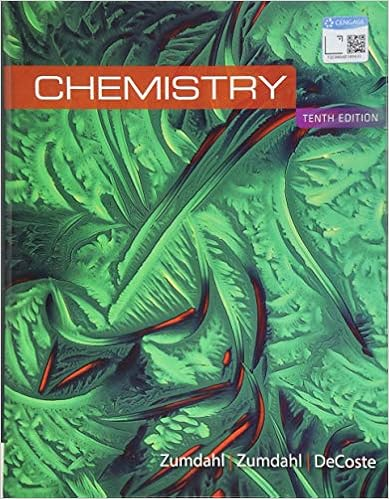# How many grams of nacl are required to precipitate

• Homework Help
• olubusayo
• 12
• 100% (1) 1 out of 1 people found this document helpful

This preview shows page 6 - 11 out of 12 pages.

##### We have textbook solutions for you!
The document you are viewing contains questions related to this textbook.The document you are viewing contains questions related to this textbook.
Chapter 4 / Exercise 3
Chemistry
ZumdahlExpert Verified
8.How many grams of NaCl are required to precipitate most of the Ag+ions from 2.50 × 102mL of 0.0113 M AgNO3solution? Write the net ionic equation for the reaction. (5 points)(Reference: Chang 4.53)6Copyright © 2014 by Thomas Edison State College. All rights reserved.
##### We have textbook solutions for you!
The document you are viewing contains questions related to this textbook.The document you are viewing contains questions related to this textbook.
Chapter 4 / Exercise 3
Chemistry
ZumdahlExpert Verified
9.What volume of 0.416M Mg(NO3)2should be added to 255 mL of 0.102 M KNO3to produce a solution with a concentration of 0.278 M NO3ions? Assume volumes are additive. (4 points)(Reference: Chang 4.69)10. Describe how to prepare 1.00 L of 0.646 MHCl solution, starting with 2.00 MHCl solution. (4 points)(Reference: Chang 4.73)7Copyright © 2014 by Thomas Edison State College. All rights reserved.
11. Calculate the volume in mL of a 1.420 MNaOH solution required to titrate the following solutions: (9 points)a.25.00 mL of a 2.430 MHCl solutionb.25.00 mL of a 4.500 MH2SO4solutionc.25.00 mL of a 1.500 MH3PO4solution (Reference: Chang 4.91)8Copyright © 2014 by Thomas Edison State College. All rights reserved.
12. A 325 mL sample of solution contains 25.3 g of CaCl2. (4 points)a.Calculate the molar concentration of Clin the solution. b.How many grams of Clare in 0.100 L of this solution?(Reference: Chang 4.148)9Copyright © 2014 by Thomas Edison State College. All rights reserved.
13. A student mixes 100.0 mL of 0.500 MAgNO3with 100.0 mL of 0.500 MCaCl2. points)a.Write the balanced molecular equation for the reaction.b.Write the net ionic equation for the reaction.c.How many grams of precipitate will form?d.What is the concentration of Ag+, NO3, Ca2+, and Clin the final solution (assume volumes are additive).(8
•••